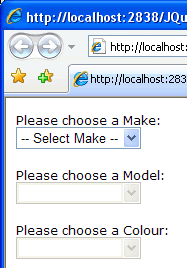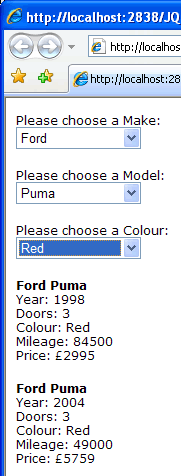# Cascading DropDownLists with jQuery and ASP.NET

Cascading DropDownLists or dependent dropdowns are the signature dish for AJAX applications. I spent quite a while fiddling to try to get some to work, before stumbling across a life-saving jQuery plugin that makes working with DropDownLists on the client-side a breeze. This article shows how it all works, and makes use of the WebService I introduced in my previous jQuery article.

The sample page for this exercise will present the user with a DropDownLis containing a selection of Makes of car. Once they have made their selection, a second DropDown will populate with available Models for the chosen Make. Selecting a Model will populate the avaialable Colours for that Model, and car details for all cars that match on Make, Model and Colour will be presented to the user when they have selected the Colour they want. For this to happen, the WebService in the previous article has been extended. Not only have I added Car objects to the List<Car>, but I have added some extra methods. Here is the code for the WebService in full:

using System;

using System.Web;

using System.Web.Services;

using System.Web.Services.Protocols;

using System.Web.Script.Services;

using System.Collections.Generic;

using System.Linq;

public class Car

{

public string Make;

public string Model;

public int Year;

public int Doors;

public string Colour;

public float Price;

public int Mileage;

}

/// <summary>

/// Summary description for CarService

/// </summary>

[WebService(Namespace = "http://tempuri.org/")]

[WebServiceBinding(ConformsTo = WsiProfiles.BasicProfile1_1)]

[ScriptService]

public class CarService : WebService

{

List<Car> Cars = new List<Car>{

new Car{Make="Audi",Model="A4",Year=1995,Doors=4,Colour="Red",Price=2995f,Mileage=122458},

new Car{Make="Ford",Model="Focus",Year=2002,Doors=5,Colour="Black",Price=3250f,Mileage=68500},

new Car{Make="BMW",Model="5 Series",Year=2006,Doors=4,Colour="Grey",Price=24950f,Mileage=19500},

new Car{Make="Renault",Model="Laguna",Year=2000,Doors=5,Colour="Red",Price=3995f,Mileage=82600},

new Car{Make="Toyota",Model="Previa",Year=1998,Doors=5,Colour="Green",Price=2695f,Mileage=72400},

new Car{Make="Mini",Model="Cooper",Year=2005,Doors=2,Colour="Grey",Price=9850f,Mileage=19800},

new Car{Make="Mazda",Model="MX 5",Year=2003,Doors=2,Colour="Silver",Price=6995f,Mileage=51988},

new Car{Make="Ford",Model="Fiesta",Year=2004,Doors=3,Colour="Red",Price=3759f,Mileage=50000},

new Car{Make="Honda",Model="Accord",Year=1997,Doors=4,Colour="Silver",Price=1995f,Mileage=99750},

new Car{Make="Audi",Model="A6",Year=2005,Doors=5,Colour="Silver",Price=22995f,Mileage=25400},

new Car{Make="Jaguar",Model="XJS",Year=1992,Doors=4,Colour="Green",Price=3450,Mileage=92000},

new Car{Make="Jaguar",Model="X Type",Year=2006,Doors=4,Colour="Grey",Price=9950f,Mileage=17000},

new Car{Make="Renault",Model="Megane",Year=2007,Doors=5,Colour="Red",Price=8995f,Mileage=8500},

new Car{Make="Peugeot",Model="406",Year=2003,Doors=4,Colour="Grey",Price=3450f,Mileage=86000},

new Car{Make="Mini",Model="Cooper S",Year=2008,Doors=2,Colour="Black",Price=14850f,Mileage=9500},

new Car{Make="Mazda",Model="5",Year=2006,Doors=5,Colour="Silver",Price=6940f,Mileage=53500},

new Car{Make="Vauxhall",Model="Vectra",Year=2007,Doors=5,Colour="White",Price=13750f,Mileage=31000},

new Car{Make="Ford",Model="Puma",Year=1998,Doors=3,Colour="Silver",Price=2995f,Mileage=84500},

new Car{Make="Ford",Model="Ka",Year=2004,Doors=3,Colour="Red",Price=2995f,Mileage=61000},

new Car{Make="Ford",Model="Focus",Year=2007,Doors=5,Colour="Blue",Price=9950f,Mileage=19000},

new Car{Make="BMW",Model="3 Series",Year=2001,Doors=4,Colour="White",Price=5950f,Mileage=98000},

new Car{Make="Citroen",Model="C5",Year=2005,Doors=5,Colour="Silver",Price=5995f,Mileage=38400},

new Car{Make="Toyota",Model="Corolla T3",Year=2004,Doors=5,Colour="Blue",Price=5995f,Mileage=71000},

new Car{Make="Toyota",Model="Yaris",Year=2005,Doors=3,Colour="Grey",Price=5350f,Mileage=39000},

new Car{Make="Porsche",Model="911",Year=2003,Doors=2,Colour="Red",Price=16995f,Mileage=88000},

new Car{Make="Ford",Model="Fiesta",Year=2004,Doors=3,Colour="Red",Price=5759f,Mileage=49000},

new Car{Make="Honda",Model="Accord",Year=1996,Doors=4,Colour="Black",Price=1995f,Mileage=105000},

new Car{Make="Audi",Model="A3 Avant",Year=2005,Doors=5,Colour="Blue",Price=12995f,Mileage=22458},

new Car{Make="Ford",Model="Mondeo",Year=2007,Doors=5,Colour="Gold",Price=12250f,Mileage=8500},

new Car{Make="BMW",Model="1 Series",Year=2006,Doors=4,Colour="Black",Price=16950f,Mileage=19500},

new Car{Make="Renault",Model="Clio",Year=2005,Doors=3,Colour="Red",Price=5995f,Mileage=32600},

new Car{Make="Toyota",Model="Verso",Year=2008,Doors=5,Colour="White",Price=12995f,Mileage=5800},

new Car{Make="Mini",Model="Cooper",Year=2003,Doors=2,Colour="Black",Price=7950f,Mileage=36800},

new Car{Make="Mazda",Model="6",Year=2007,Doors=4,Colour="Blue",Price=16995f,Mileage=11300},

new Car{Make="Ford",Model="Mondeo",Year=2004,Doors=5,Colour="Green",Price=8759f,Mileage=66000},

new Car{Make="Honda",Model="Civic",Year=1997,Doors=4,Colour="Grey",Price=1995f,Mileage=99750},

new Car{Make="Audi",Model="Q7",Year=2005,Doors=5,Colour="Black",Price=22995f,Mileage=25400},

new Car{Make="Jaguar",Model="XK8",Year=1992,Doors=4,Colour="Blue",Price=3450,Mileage=92000},

new Car{Make="Jaguar",Model="S Type",Year=2006,Doors=4,Colour="Red",Price=9950f,Mileage=17000},

new Car{Make="Renault",Model="Megane",Year=2007,Doors=5,Colour="Yellow",Price=8995f,Mileage=8500},

new Car{Make="Peugeot",Model="406",Year=2003,Doors=4,Colour="White",Price=3450f,Mileage=86000},

new Car{Make="Mini",Model="Cooper",Year=2008,Doors=2,Colour="Red",Price=14850f,Mileage=9500},

new Car{Make="Mazda",Model="5",Year=2006,Doors=5,Colour="White",Price=6940f,Mileage=53500},

new Car{Make="Vauxhall",Model="Vectra",Year=2007,Doors=5,Colour="Blue",Price=13750f,Mileage=31000},

new Car{Make="Ford",Model="Puma",Year=1998,Doors=3,Colour="Red",Price=2995f,Mileage=84500},

new Car{Make="Ford",Model="Ka",Year=2004,Doors=3,Colour="Black",Price=2995f,Mileage=61000},

new Car{Make="Ford",Model="Focus",Year=2007,Doors=5,Colour="Grey",Price=9950f,Mileage=19000},

new Car{Make="BMW",Model="3 Series",Year=2001,Doors=4,Colour="Red",Price=5950f,Mileage=98000},

new Car{Make="Citroen",Model="C5",Year=2005,Doors=5,Colour="Yellow",Price=5995f,Mileage=38400},

new Car{Make="Toyota",Model="Corolla T3",Year=2004,Doors=5,Colour="Red",Price=5995f,Mileage=71000},

new Car{Make="Toyota",Model="Yaris",Year=2005,Doors=3,Colour="Black",Price=5350f,Mileage=39000},

new Car{Make="Porsche",Model="911",Year=2003,Doors=2,Colour="White",Price=16995f,Mileage=88000},

new Car{Make="Ford",Model="Fiesta",Year=2004,Doors=3,Colour="Grey",Price=5759f,Mileage=49000},

new Car{Make="Honda",Model="Accord",Year=1996,Doors=4,Colour="Green",Price=1995f,Mileage=105000}

};

[WebMethod]

public List<Car> GetCarsByDoors(int doors)

{

var query = from c in Cars

where c.Doors == doors

select c;

return query.ToList();

}

[WebMethod]

public List<Car> GetAllCars()

{

return Cars;

}

[WebMethod]

public List<string> GetCarMakes()

{

var query = (from c in Cars

orderby c.Make

select c.Make).Distinct();

return query.ToList();

}

[WebMethod]

public List<string> GetCarsByModel(string make)

{

var query = (from c in Cars

where c.Make == make

orderby c.Model

select c.Model).Distinct();

return query.ToList();

}

[WebMethod]

public List<string> GetCarsByColour(string make, string model)

{

var query = (from c in Cars

where c.Make == make && c.Model == model

orderby c.Colour

select c.Colour).Distinct();

return query.ToList();

}

[WebMethod]

public List<Car> GetCarListByColour(string make, string model, string colour)

{

var query = from c in Cars

where (c.Make == make &&

c.Model == model &&

c.Colour == colour)

select c;

return query.ToList();

}

}

Three of the additional methods return types of List<string>. These are used to populate the DropDownLists. The final method retrieves a List<Car> that meets the Model, Make and Colour criteria that have been selected using the DropDownLists. I say "DropDownLists", but actually there is only one in the aspx file. The other two are straightforward HTML <select> elements. It's generally good practice to only use ASP.NET Server-Side controls where they are actually needed. Since all the work in populating and referencing the second and third list is done on the client, I don't need to reference them from Code-Behind. Consequently, there is no need to have runat="server" controls.

<form id="form1" runat="server">

<div>

<div>

<p>

<label>

<asp:DropDownList ID="ddlMake" runat="server" />

</p>

</div>

<div>

<p>

<label>

<select id="ddlModel">

</select></p>

</div>

<div>

<p>

<label>

<select id="ddlColour">

</select></p>

</div>

<div id="output"></div>

</div>

</form>

The server control DropDownList will be filled from code-behind on Page_Load:

protected void Page_Load(object sender, EventArgs e)

{

if (!IsPostBack)

{

CarService service = new CarService();

List<string> Makes = service.GetCarMakes();

ddlMake.DataSource = Makes;

ddlMake.DataBind();

ddlMake.Items.Insert(0, " -- Select Make -- ");

}

}

Whenever populating a DropDownList from code-behind and adding a default first option, you should always make sure that the page hasn't been posted back when doing so. Otherwise the default first option will be added every time there's a postback. It would be perfectly possible to load this DropDownList using AJAX and make it a <select> element instead. But that would just be using AJAX for the sake of it, rather than because there is an identifiable need.

Having said that, AJAX is what this example is all about, so let's get into the client-side code:

<script src="script/jquery-1.2.6.min.js" type="text/javascript"></script>

<script src="script/jquery.selectboxes.min.js" type="text/javascript"></script>

<script type="text/javascript">

\$(function() {

\$('#ddlMake').change(getModels);

\$('#ddlModel').attr('disabled', true);

\$('#ddlColour').attr('disabled', true);

});

Two external script files are referenced: the first is the condensed version of jQuery, while the second is the plugin for managing dropdowns or selects. This is followed by some code that will run in the onload event of the page. It applies an event handler to the onchange event of the "Make" list which calls the getModels() function, and it also sets the second and third selects to disabled.Choosing an option in the first list will fire the getModels() function which is as follows:

function getModels() {

\$.ajax({

type: "POST",

url: "CarService.asmx/GetCarsByModel",

data: "{make: '" + \$('#ddlMake').val() + "'}",

contentType: "application/json; charset=utf-8",

dataType: "json",

success: function(response) {

var models = (typeof response.d) == 'string' ? eval('(' + response.d + ')') : response.d;

\$('#ddlModel').attr('disabled', false).change(getColours).removeOption(/./).addOption('', ' -- Select Model -- ');

\$('#ddlColour').attr('disabled', true).removeOption(/./);

for (var i = 0; i < models.length; i++) {

var val = models[i];

var text = models[i];

}

}

});

}

This AJAX call follows a very similar pattern to the ones introduced in my previous article. It calls a WebMethod called GetCarsByModel, with the Make passed as a parameter. Once the response has been received and validated, a number of things happen. The Model select list is enabled, and jQuery chaining is used to first disable the Colour dropdown (in case it was enabled by a previous Make selection, and then add an onchange event handler which fires the getColours() function. Next the dropdown is cleared using the SelectBoxes plugin's removeOption() function, and finally a default option is added to the dropdownlist. All of this on one line.

The Colour dropdown is then treated to chained commands that disable, then clear it of any previous options. Finally the response is iterated and added as option elements to the Model dropdown. As opposed to the response delivered by the WebMethods in the previous article, this response is an object called d whose single property simply contains an array of strings. For example, if Ford is selected from the Make dropdown, the response is as follows:

{"d":["Fiesta","Focus","Ka","Mondeo","Puma"]}

Selecting one of these models will cause the onchange event handler to fire, which calls the getColours() function:

function getColours() {

\$.ajax({

type: "POST",

url: "CarService.asmx/GetCarsByColour",

data: "{make: '" + \$('#ddlMake').val() + "', model: '" + \$('#ddlModel').val() + "'}",

contentType: "application/json; charset=utf-8",

dataType: "json",

success: function(response) {

var colours = (typeof response.d) == 'string' ? eval('(' + response.d + ')') : response.d;

\$('#ddlColour').attr('disabled', false).change(getCarListByColour).removeOption(/./).addOption('', ' -- Select Colour -- ');

for (var i = 0; i < colours.length; i++) {

var val = colours[i];

var text = colours[i];

}

}

});

}

Two parameters are passed into the call to the WebMethod in this function, these being the Make dropdown selected value and the Model dropdown selected value. Aside from that the rest of the code should be familiar form the previous function. Notice again the jQuery chaining being employed to enable the Colour dropdown, add an onchange event handler, clear it and finally set a default option. The onchange event handler calls the final function, which results in the collection of cars being retrieved from the Web Service and then displayed in the final div on the page:

function getCarListByColour() {

\$.ajax({

type: "POST",

url: "CarService.asmx/GetCarListByColour",

data: "{make: '" + \$('#ddlMake').val() + "', " +

"model: '" + \$('#ddlModel').val() + "', " +

"colour: '" + \$('#ddlColour').val() + "'}",

contentType: "application/json; charset=utf-8",

dataType: "json",

success: function(response) {

var cars = (typeof response.d) == 'string' ? eval('(' + response.d + ')') : response.d;

\$('#output').empty();

for (var i = 0; i < cars.length; i++) {

\$('#output').append('<p><strong>' + cars[i].Make + ' ' +

cars[i].Model + '</strong><br /> Year: ' +

cars[i].Year + '<br />Doors: ' +

cars[i].Doors + '<br />Colour: ' +

cars[i].Colour + '<br />Mileage: ' +

cars[i].Mileage + '<br />Price: £' +

cars[i].Price + '</p>');

}

}

});

}

</script>

If you read my previous article on calling Web Services wtih jQuery, there is nothing here that is new. The script simply calls the GetCarListByColour() WebMethod and returns an array of objects as a JSON string. The Cars that are returned are those that match the Make, Model and Colours selected in the DropDowns and the result is written to the div at the bottom of the page.Using jQuery on its own for this task would have required a lot more work, but digging around the host of plugins available for jQuery sped the whole process up dramatically.